Main MRPT website > C++ reference for MRPT 1.5.7
data_utils.h File Reference
#include <mrpt/utils/utils_defs.h>
#include <mrpt/math/wrap2pi.h>
#include <mrpt/math/CMatrixFixedNumeric.h>
#include <mrpt/math/ops_matrices.h>
Include dependency graph for data_utils.h: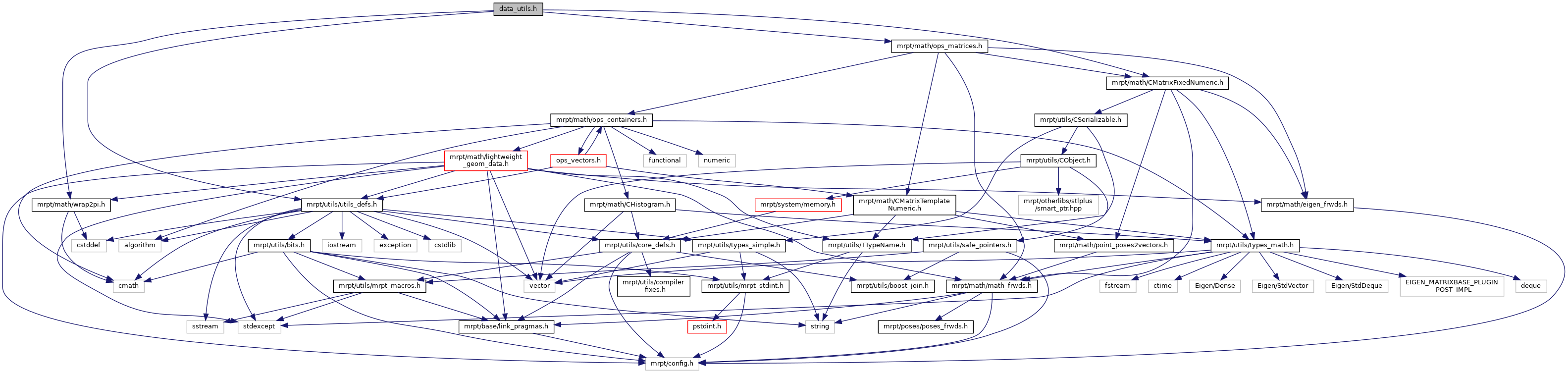This graph shows which files directly or indirectly include this file: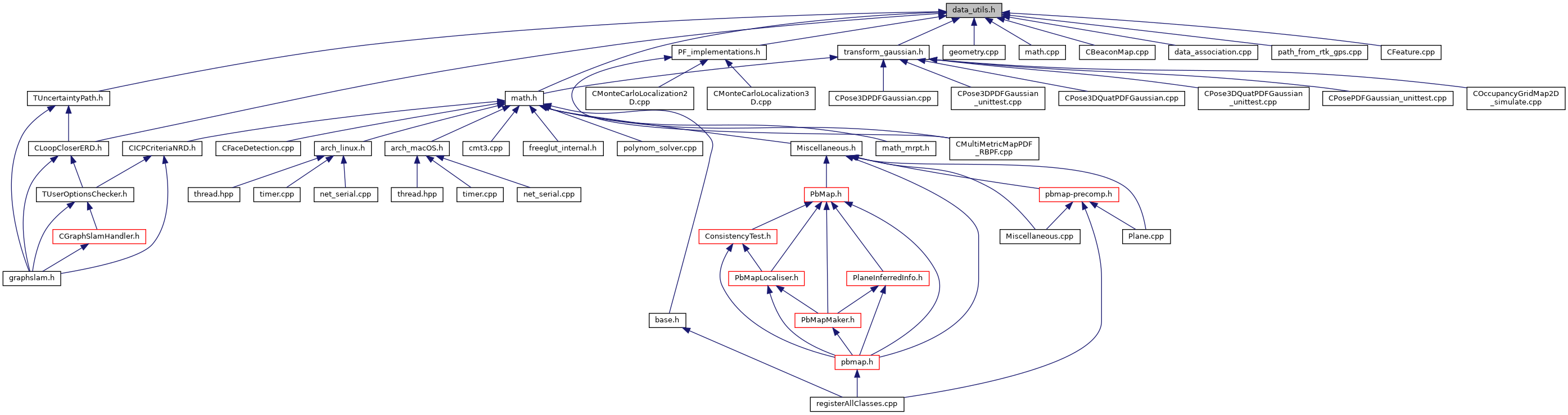Go to the source code of this file.

## Namespaces

mrpt
This is the global namespace for all Mobile Robot Programming Toolkit (MRPT) libraries.

mrpt::math
This base provides a set of functions for maths stuff.

## Functions

Probability density distributions (pdf) distance metrics
template<class VECTORLIKE1 , class VECTORLIKE2 , class MAT >
MAT::Scalar mrpt::math::mahalanobisDistance2 (const VECTORLIKE1 &X, const VECTORLIKE2 &MU, const MAT &COV)
Computes the squared mahalanobis distance of a vector X given the mean MU and the covariance inverse COV_inv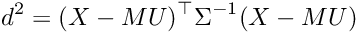template<class VECTORLIKE1 , class VECTORLIKE2 , class MAT >
VECTORLIKE1::Scalar mrpt::math::mahalanobisDistance (const VECTORLIKE1 &X, const VECTORLIKE2 &MU, const MAT &COV)
Computes the mahalanobis distance of a vector X given the mean MU and the covariance inverse COV_invtemplate<class VECTORLIKE , class MAT1 , class MAT2 , class MAT3 >
MAT1::Scalar mrpt::math::mahalanobisDistance2 (const VECTORLIKE &mean_diffs, const MAT1 &COV1, const MAT2 &COV2, const MAT3 &CROSS_COV12)
Computes the squared mahalanobis distance between two non-independent Gaussians, given the two covariance matrices and the vector with the difference of their means. More...

template<class VECTORLIKE , class MAT1 , class MAT2 , class MAT3 >
VECTORLIKE::Scalar mrpt::math::mahalanobisDistance (const VECTORLIKE &mean_diffs, const MAT1 &COV1, const MAT2 &COV2, const MAT3 &CROSS_COV12)
Computes the mahalanobis distance between two non-independent Gaussians (or independent if CROSS_COV12=NULL), given the two covariance matrices and the vector with the difference of their means. More...

template<class VECTORLIKE , class MATRIXLIKE >
MATRIXLIKE::Scalar mrpt::math::mahalanobisDistance2 (const VECTORLIKE &delta_mu, const MATRIXLIKE &cov)
Computes the squared mahalanobis distance between a point and a Gaussian, given the covariance matrix and the vector with the difference between the mean and the point. More...

template<class VECTORLIKE , class MATRIXLIKE >
MATRIXLIKE::Scalar mrpt::math::mahalanobisDistance (const VECTORLIKE &delta_mu, const MATRIXLIKE &cov)
Computes the mahalanobis distance between a point and a Gaussian, given the covariance matrix and the vector with the difference between the mean and the point. More...

template<typename T >
mrpt::math::productIntegralTwoGaussians (const std::vector< T > &mean_diffs, const CMatrixTemplateNumeric< T > &COV1, const CMatrixTemplateNumeric< T > &COV2)
Computes the integral of the product of two Gaussians, with means separated by "mean_diffs" and covariances "COV1" and "COV2". More...

template<typename T , size_t DIM>
mrpt::math::productIntegralTwoGaussians (const std::vector< T > &mean_diffs, const CMatrixFixedNumeric< T, DIM, DIM > &COV1, const CMatrixFixedNumeric< T, DIM, DIM > &COV2)
Computes the integral of the product of two Gaussians, with means separated by "mean_diffs" and covariances "COV1" and "COV2". More...

template<typename T , class VECLIKE , class MATLIKE1 , class MATLIKE2 >
void mrpt::math::productIntegralAndMahalanobisTwoGaussians (const VECLIKE &mean_diffs, const MATLIKE1 &COV1, const MATLIKE2 &COV2, T &maha2_out, T &intprod_out, const MATLIKE1 *CROSS_COV12=NULL)
Computes both, the integral of the product of two Gaussians and their square Mahalanobis distance. More...

template<typename T , class VECLIKE , class MATRIXLIKE >
void mrpt::math::mahalanobisDistance2AndLogPDF (const VECLIKE &diff_mean, const MATRIXLIKE &cov, T &maha2_out, T &log_pdf_out)
Computes both, the logarithm of the PDF and the square Mahalanobis distance between a point (given by its difference wrt the mean) and a Gaussian. More...

template<typename T , class VECLIKE , class MATRIXLIKE >
void mrpt::math::mahalanobisDistance2AndPDF (const VECLIKE &diff_mean, const MATRIXLIKE &cov, T &maha2_out, T &pdf_out)
Computes both, the PDF and the square Mahalanobis distance between a point (given by its difference wrt the mean) and a Gaussian. More...

template<class VECTOR_OF_VECTORS , class MATRIXLIKE , class VECTORLIKE , class VECTORLIKE2 , class VECTORLIKE3 >
void mrpt::math::covariancesAndMeanWeighted (const VECTOR_OF_VECTORS &elements, MATRIXLIKE &covariances, VECTORLIKE &means, const VECTORLIKE2 *weights_mean, const VECTORLIKE3 *weights_cov, const bool *elem_do_wrap2pi=NULL)
Computes covariances and mean of any vector of containers, given optional weights for the different samples. More...

template<class VECTOR_OF_VECTORS , class MATRIXLIKE , class VECTORLIKE >
void mrpt::math::covariancesAndMean (const VECTOR_OF_VECTORS &elements, MATRIXLIKE &covariances, VECTORLIKE &means, const bool *elem_do_wrap2pi=NULL)
Computes covariances and mean of any vector of containers. More...

template<class VECTORLIKE1 , class VECTORLIKE2 >
void mrpt::math::weightedHistogram (const VECTORLIKE1 &values, const VECTORLIKE1 &weights, float binWidth, VECTORLIKE2 &out_binCenters, VECTORLIKE2 &out_binValues)
Computes the weighted histogram for a vector of values and their corresponding weights. More...

template<class VECTORLIKE1 , class VECTORLIKE2 >
void mrpt::math::weightedHistogramLog (const VECTORLIKE1 &values, const VECTORLIKE1 &log_weights, float binWidth, VECTORLIKE2 &out_binCenters, VECTORLIKE2 &out_binValues)
Computes the weighted histogram for a vector of values and their corresponding log-weights. More...

double BASE_IMPEXP mrpt::math::averageLogLikelihood (const CVectorDouble &logLikelihoods)
A numerically-stable method to compute average likelihood values with strongly different ranges (unweighted likelihoods: compute the arithmetic mean). More...

double BASE_IMPEXP mrpt::math::averageWrap2Pi (const CVectorDouble &angles)
Computes the average of a sequence of angles in radians taking into account the correct wrapping in the range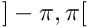, for example, the mean of (2,-2) is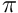, not 0. More...

double BASE_IMPEXP mrpt::math::averageLogLikelihood (const CVectorDouble &logWeights, const CVectorDouble &logLikelihoods)
A numerically-stable method to average likelihood values with strongly different ranges (weighted likelihoods). More...

 Page generated by Doxygen 1.8.14 for MRPT 1.5.7 Git: 8277875f6 Mon Jun 11 02:47:32 2018 +0200 at lun oct 28 01:50:49 CET 2019